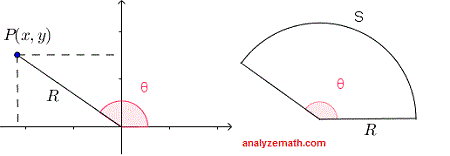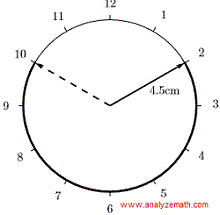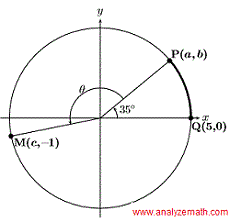# Circles, Sectors and Trigonometry Problems with Solutions and Answers

Sectors and Circles trigonometry problems are presented along with their detailed solutions.
When solving the problems below, we will make use of the definition of the trigonometric functions of angle θ in terms of the coordinates of point P(x , y) that is on the terminal side of an angle θ.
cos(θ) = x / R     tan(θ) = y / x     sec(θ) = R / x
sin(θ) = y / R     cot(θ) = x / y     CSC(θ) = R / y
R = √(x2 + y2)
We will also use the relationship between the arc length S, the radius R and the central angle θ of a sector: S = R θ     (θ in RADIANS).

## Problems on Circles and Arcs

1. If the coordinates of point A, in the circle below, are (8, 0) and arc s has a length of 20 units, find the coordinates of point P in the diagram below..

Solution
Radius R of the given circle is equal to 8. We first use the formula for the arc s in terms of the radius R and the angle θ to find θ in radians.
s = R θ
2 0 = 8 θ
θ = 20 / 8 = 2.5 radians
We now use the definitions for sine and cosine to find the coordinates x and y of P.
cos(θ) = x / R     and     sin(θ) = y / R
cos(θ) = x / R     gives     x = R cos(θ) = 8 cos(2.5) = -6.40
sin(θ) = y / R     gives     y = R sin(θ) = 8 sin(2.5) = 4.79
Point P has the coordinates (-6.40 , 4.79).

2. In a rectangular coordinate system, a circle with its center at the origin passes through the point(4√2 , 5√2).
What is the length of the arc S?.

Solution
We use the definiton for tangent to find angle θ.
tan(θ) = y / x = 5√2 / 4√2 = 5 / 4
θ = arctan(5 / 4)
Use fromula for s in terms of θ and the radius R.
S = R θ = √ (4√2)2 + (5√2)2 arctan(5 / 4) ≈ 8.11

3. The length of the minutes hand in a clock is 4.5 cm. Find the length of the arc traced by the end of the minutes hand between 11:10 pm and 11:50 pm..

Solution
From 11:10 to 11:50, there
11:50 - 11:10 = 40 minutes
One complete rotation of the minutes hand corresponds to 60 minutes and to an angle of 360�. We therefore can write that there are
360 � / 60 minutes = 6 � / minutes
If θ is the central angle corresponding to the arc traced by the minutes hand, then it is given by
θ = (6 �/ minute) � 40 minutes = 240 �
The arc S traced is then given by
S = R θ , (θ in radians).
S = R θ = 4.5 cm 240 � π / 180 = 6π

4. The points P(a , b), Q(5 , 0) and M(c , -1) are located on the circle with the center O(0 , 0) and radius R of 5 units as shown below. Calculate the:
a) The coordinates (a,b) of point P.
b) The arc length between Q and P in counterclockwise direction.
c) The x-coordinate c of point M.
d) Angle θ..

Solution
a) Point P is the terminal side of an angle in standard position of size 35�. Hence
a = R cos (35�) = 5 cos (35�)     and     b = R sin(35�) = 5 sin (35�)
b) The central angle QOP is known, therefore the length of the arc PQ is given by
arc PQ = r�35 � π / 180 = 5�35 � π / 180 ≈ 3.05 units
c) Point C is on the circle, therefore the distance from the center of the circle (0,0) to point C is equal to the radius.
(c - 0)2 + (-1 - 0)2 = 5
Square both sides of the above equation and solve for c.
(c - 0)2 + (-1 - 0)2 = 25
c = ~+mn~ √ 24 = ~+mn~ 2√ 6
Point M is in quadrant III and therefore c is negative. Hence point M has the coordinates
M(- 2√ 6, -1)
d) Point M is on the terminal side of angle θ+35�. Hence using the definition of the tangent of an angle in standard position in terms of the coordinates of a point on the terminal side, we have
tan(θ + 35 �) = 1 / 2√ 6
Point M is in quadarnt III, therefore θ + 35 � is greater than 180� and therefore
θ + 35 � = 180 + arctan(1 / 2√ 6)
θ = 180 + arctan(1 / 2√ 6) - 35 � = 156.54�

5. Circle of radius R = 4 units and center O is shown below.
a) Find the length of the arc between D and P.
b) Find the coordinates (a,b) of P.
c) Find the length of segment PD,..

Solution
a) The central angle DOP is known, therefore length of the arc DP is given by (do not forget to convert degrees to radians)
arc PQ = R 130 π / 180 = 4 � 130 π / 180 ≈ 9.08 units
b) Point P is on the terminal side of an angle in standard position. Therefore the coordinates are given by:
a = R cos(130�) = 4 cos(130�)     and     b = R sin(130�) = 4 sin(130�)
c) Use the distance formula between two points P and D to find the length of the line segment PD.
PD = √ (a - 4)2 + (b - 0)2
= √ (4 cos(130�) - 4)2 + (4 sin(130�) - 0)2 ≈ 7.25

6. In the circle of center O and radius R = 3 shown below, the length of the arc S between A and B is 12.5 units. Find the coordinates (a , b) of point B..

Solution
We first need to find the size θ of the central angle AOB.
S = R θ
θ = S / R = 12.5 / 3 radians
We now calculate the coordinates a and b of point B.
a = R cosθ = 3 cos(12.5 / 3)
b = R cosθ = 3 sin(12.5 / 3)

7. Point P has coordinates (4.1 , b) and is located on the circle, of center O and radius R = 5, in quadrant I. Find the length of arc S..

Solution
We first need to calculate the size θ of the central angle DOP using the x coordinate of point P and the radius.
4.1 = 5 cos(θ)
cos(θ) = 4.1 / 5
θ = arccos(4.1 / 5)
We calculate the arc S.
S = R θ = 5 arccos(4.1 / 5)